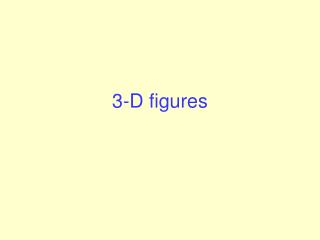DownloadDownload Presentation3-D figures

# 3-D figures

Télécharger la présentation## 3-D figures

- - - - - - - - - - - - - - - - - - - - - - - - - - - E N D - - - - - - - - - - - - - - - - - - - - - - - - - - -
##### Presentation Transcript

1. 3-D figures

2. Reflectional Symmetry For 2D figure: If a plane figure can be divided by a line into two identical parts and these parts are mirror images of each other, then we say that the figure has reflectional symmetry. axis of symmetry->

3. Reflectional Symmetry For 3D- figure: If a solid is evenly divided into two parts by a plane, the two parts are the mirror images of each other. This solid is said to have the property of reflectional symmetry and the plane is called the plane of reflection.

4. Cube How many planes of reflection of a cube? There are totally 9 planes of reflection for a cube

5. 5 4 6 3 1 2 Tetrahedron How many planes of reflection of a tetrahedron? There are totally 6 planes of reflection for a tetrahedron

6. Rotational symmetry • If a solid coincides with itself n times (where n  2) when it is rotated one revolution about an axis inside the solid, the solid is said to have n-fold rotational symmetry about the axis, and the axis is called an axis of rotation. • Note: Cube (3D) has rotational symmetry but square (2D) has not

7. Cube How many axes rotational symmetry axes of a cube? There are 13 rotational Symmetry axes

8. Tetrahedron How many axes rotational symmetry axes of a tetrahedron? There are 7 Rotational symmetry axes

9. Orthographical Projection An orthographic projection uses three plane figures to illustrate the shape of a solid. These plane figures show the front view, the side view and the top view of the solid. Front view - viewing the object from the front. Side view - viewing the object from the side. Top view - viewing the object from the top.

10. Front view : Side view : Top view : Pyramid

11. Nets of solids A net is a plane figure that can be folded into a solid.

12. Euler Formula V: number of vertices = 4 E: number of edges = 6 F: number of faces = 4 V- E + F = 2

13. END